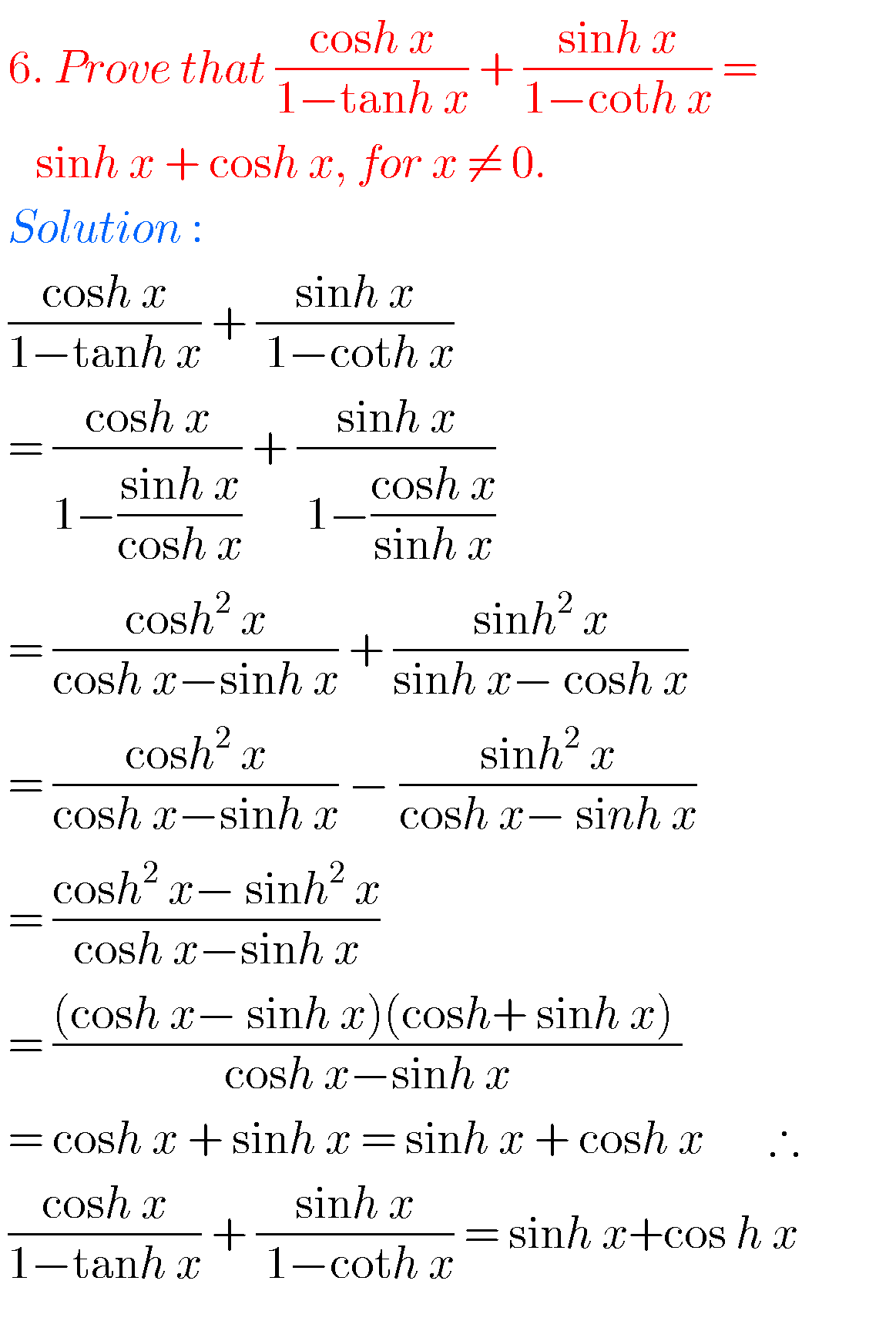## Hyperbolic Functions exercise 9(a) solutions Inter Maths 1A

HYPERBOLIC FUNCTIONS Solutions Inter Maths 1B Inter first year Maths 1A textbook chapter 9 Hyperbolic Functions exercise 9(a) solutions are given. You study textbook lesson Hyperbolic functons very well. Observe the example problems and solutions given in the textbook. Observe the given below solutions and try them in your own method. You can also see …